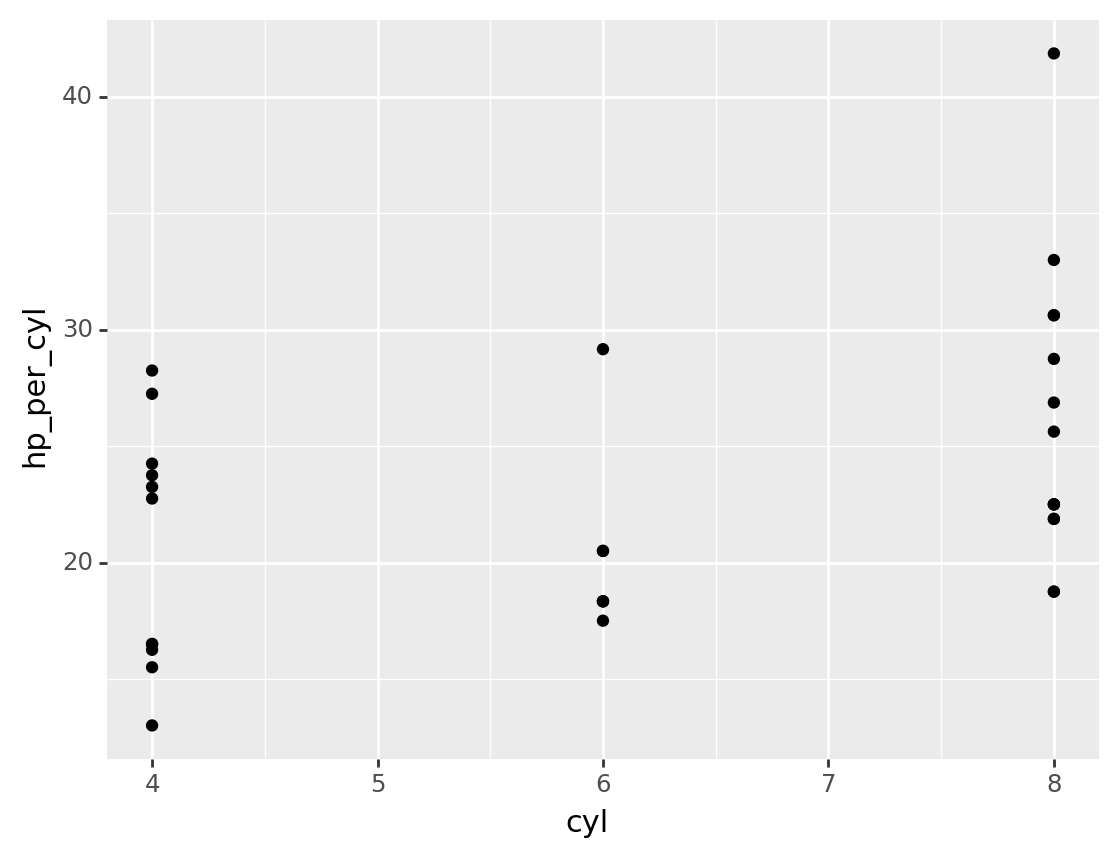Siuba is a tool for concise, flexible data-analysis over multiple data sources. It currently supports pandas DataFrames and SQL tables.

## Installing

``pip install siuba``

## Basic use

The code below uses the example DataFrame mtcars, to get the average horsepower (hp) per cylinder.

``````from siuba import _, group_by, summarize
from siuba.data import mtcars

(mtcars
>> group_by(_.cyl)
>> summarize(avg_hp = _.hp.mean())
)``````
cyl avg_hp
0 4 82.636364
1 6 122.285714
2 8 209.214286

There are three key concepts in this example:

concept example meaning
verb `group_by(...)` a function that operates on a table, like a DataFrame or SQL table
lazy expression `_.hp.mean()` an expression created with `siuba._`, that represents actions you want to perform
pipe `mtcars >> group_by(...)` a syntax that allows you to chain verbs with the `>>` operator

## Lazy expressions (`_`)

A siu expression is a way of specifying what action you want to perform. This allows siuba verbs to decide how to execute the action, depending on whether your data is a local DataFrame or remote table.

``````from siuba import _

_.cyl == 4``````
``````█─==
├─█─.
│ ├─_
│ └─'cyl'
└─4``````

Notice how the output represents each step in our lazy expression, with these pieces:

• black box █ - a method like checking equality (`==`) or getting an attribute (`.`).
• underscore (`_`) - a placeholder for a table of data.

We can use these expressions like lambda functions. For example, to keep specific rows of a pandas DataFrame.

``````# old approach: repeat name
mtcars[mtcars.cyl == 4]

# old approach: lambda
mtcars[lambda _: _.cyl == 4]

# siu approach
mtcars[_.cyl == 4]``````
mpg cyl disp hp drat wt qsec vs am gear carb
2 22.8 4 108.0 93 3.85 2.320 18.61 1 1 4 1
7 24.4 4 146.7 62 3.69 3.190 20.00 1 0 4 2
... ... ... ... ... ... ... ... ... ... ... ...
27 30.4 4 95.1 113 3.77 1.513 16.90 1 1 5 2
31 21.4 4 121.0 109 4.11 2.780 18.60 1 1 4 2

11 rows × 11 columns

Note that like the lambda function, siuba avoids typing the same (potentially_very_long) name twice, while also being a bit shorter.

## Table verbs

Verbs are functions that operate on a table of data. They can be combined using a pipe with the `>>` operator.

``````from siuba import _, mutate, filter, group_by, summarize
from siuba.data import mtcars``````

### Mutate

The previous example can be re-written in siuba as the following.

``````(mtcars
>> group_by(_.cyl)
>> mutate(demeaned = _.hp - _.hp.mean())
)``````

(grouped data frame)

mpg cyl disp hp drat wt qsec vs am gear carb demeaned
0 21.0 6 160.0 110 3.90 2.620 16.46 0 1 4 4 -12.285714
1 21.0 6 160.0 110 3.90 2.875 17.02 0 1 4 4 -12.285714
... ... ... ... ... ... ... ... ... ... ... ... ...
30 15.0 8 301.0 335 3.54 3.570 14.60 0 1 5 8 125.785714
31 21.4 4 121.0 109 4.11 2.780 18.60 1 1 4 2 26.363636

32 rows × 12 columns

Note that there is a key difference: mutate returned a pandas DataFrame with the new column (demeaned) at the end. This is a core feature of siuba verbs–tables in and tables out.

### Filter

Below are examples of keeping certain rows with filter, and calculating a single number per group with summarize.

``````g_cyl = group_by(mtcars, _.cyl)

# keep lowest hp per group
g_cyl >> filter(_.hp == _.hp.min())``````

(grouped data frame)

mpg cyl disp hp drat wt qsec vs am gear carb
5 18.1 6 225.0 105 2.76 3.460 20.22 1 0 3 1
18 30.4 4 75.7 52 4.93 1.615 18.52 1 1 4 2
21 15.5 8 318.0 150 2.76 3.520 16.87 0 0 3 2
22 15.2 8 304.0 150 3.15 3.435 17.30 0 0 3 2

### Summarize

``g_cyl >> summarize(avg_hp = _.hp.mean())``
cyl avg_hp
0 4 82.636364
1 6 122.285714
2 8 209.214286

## Column operations

The verbs above received a few different calculations as arguments:

• `_.hp.mean()`
• `_.hp.min()`

You can use any methods from the underlying pandas objects as methods.

``````# outside
mtcars.shape + 1

# inside mutate
mtcars >> mutate(res = _.shape + 1)``````
mpg cyl disp hp drat wt qsec vs am gear carb res
0 21.0 6 160.0 110 3.90 2.620 16.46 0 1 4 4 33
1 21.0 6 160.0 110 3.90 2.875 17.02 0 1 4 4 33
... ... ... ... ... ... ... ... ... ... ... ... ...
30 15.0 8 301.0 335 3.54 3.570 14.60 0 1 5 8 33
31 21.4 4 121.0 109 4.11 2.780 18.60 1 1 4 2 33

32 rows × 12 columns

This includes the `str` and `dt` attribute accessor methods:

``````import pandas as pd

df = pd.DataFrame({"x": ["apple", "banana"]})

# outside
df.x.str.contains("a")

# inside mutate
df >> mutate(res = _.x.str.contains("a"))``````
x res
0 apple True
1 banana True

## Using with plotnine

Fortnuately, plotnine supports siuba’s style of piping, so is easy to plug in to!

``````from siuba import mutate, _
from plotnine import ggplot, aes, geom_point

(mtcars
>> mutate(hp_per_cyl = _.hp / _.cyl)
>> ggplot(aes("cyl", "hp_per_cyl"))
+ geom_point()
)````````<ggplot: (8761248305454)>``